# SAT Math Multiple Choice Question 270: Answer and Explanation

### Test Information

Question: 270

15.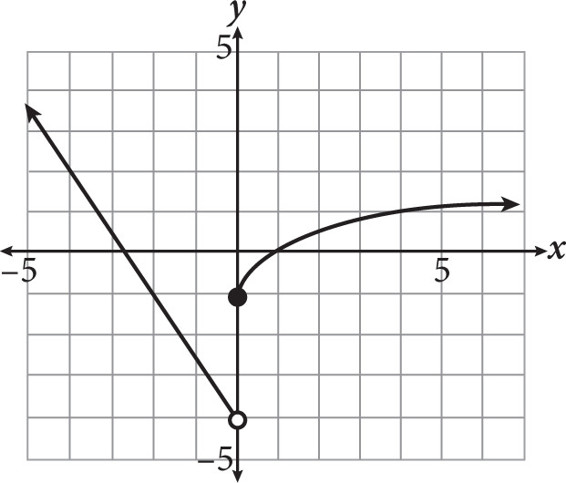Which of the following piecewise functions could have been used to generate the graph above?

• A.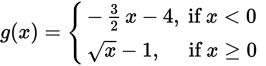• B.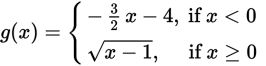• C.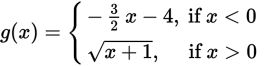• D.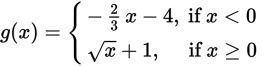Getting to the Answer: First, notice that both pieces of the graph either start or stop at 0, but one has a closed dot and the other has an open dot. This means you can eliminate C right away because the inequality symbol in both equations would lead to open dots on the graph. To choose among the remaining answers, think about parent functions and transformations. To the left of x = 0, the graph is a line with a slope of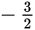and a y-intercept of -4, so you can eliminate D because the slope of the line is incorrect. Now, look to the right of x = 0-the graph is a square root function that has been moved down 1 unit, so its equation is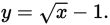This means (A) is correct. (The square root portion of C would have been moved to the left 1 unit rather than down 1.)